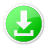**Texture Reconstruction**

=====

In this challenge, a textured plane is placed in the [standard setup](/doc/setup). The shape of the plane is known, but its texture needs to be reconstructed.

Reconstructing the texture of a plane is somewhat similar to the problem of reconstruction flat geometric objects. However, direct texture reconstruction has not yet been attempted in the literature and thus this challenges only contains the somewhat simple scenario of a single, flat plane. Once research advances, more complex shapes might be added.

Dataset
=======

The dataset contains twelve tasks with varying difficulty:

Name       | Resolution       | Pixel depth
-----------|------------------|--------------
Character  | $4 \times 4$     | $\{0, 1\}$
Digit      | $4 \times 4$     | $\{0, 1\}$
Letter     | $4 \times 4$     | $\{0, 1\}$
**Smiley** | $4 \times 4$     | $\{0, 1\}$
House      | $16 \times 16$   | $\{0, 0.25, 0.5, 0.75, 1\}$
Number     | $16 \times 16$   | $\{0, 0.25, 0.5, 0.75, 1\}$
Pattern    | $16 \times 16$   | $\{0, 0.25, 0.5, 0.75, 1\}$
**Text**   | $16 \times 16$   | $\{0, 0.25, 0.5, 0.75, 1\}$
**Books**  | $128 \times 128$ | $[0, 1]$
Concert    | $128 \times 128$ | $[0, 1]$
Fan        | $128 \times 128$ | $[0, 1]$
Industrial | $128 \times 128$ | $[0, 1]$

[](/database/Texture/Texture.7z) **Database**

For the tasks printed in bold, ground truth information is available:

[](/database/Texture/TextureGT.7z) **Ground truth images**

The ground truth images can be used to calibrate the reconstruction output to the actual pixel values.

Submission
==========
The submission should contain a png file for each task. Solutions are not restrained to the pixel depth or resolution of the reference file. Especially for the first two groups, arbitrary gray values can be used to model uncertainty.